Next: Exercises Up: Waves in Incompressible Fluids Previous: Capillary Waves at an

# Wind Driven Waves in Deep Water

Consider the scenario described in the previous section. Suppose that the lower fluid is a body of deep water at rest, and the upper fluid is the atmosphere. Let the air above the surface of the water move horizontally at the constant velocity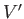. Suppose thatis the density of water,the specific gravity of air with respect to water, and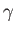the surface tension at an air/water interface. With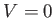,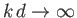,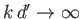, the dispersion relation (11.132) reduces to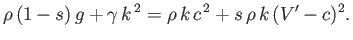(11.139)

This expression can be rearranged to give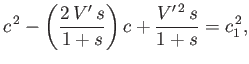(11.140)

which is a quadratic equation for the phase velocity,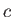, of the wave. Here,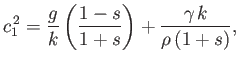(11.141)

where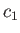is the phase velocity that the wave would have in the absence of the wind. In fact, we can write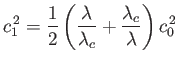(11.142)

whereis the capillary wavelength, andand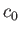are defined in Equations (11.134) and (11.135), respectively.

For a given wavelength,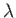, the wave velocity,, attains its maximum value,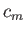, when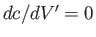. According to the dispersion relation (11.140), this occurs when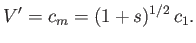(11.143)

If the wind has any other velocity, greater or less than, then the wave velocity is less than.

According to Equation (11.140), the wave velocity,, becomes complex, indicating an instability, when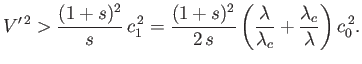(11.144)

We conclude that if the wind speed exceeds the critical value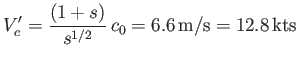(11.145)

then waves whose wavelengths fall within a certain range, centered around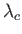, are unstable and grow to large amplitude.

The two roots of Equation (11.140) are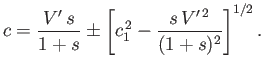(11.146)

Moreover, if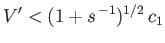(11.147)

then these roots have opposite signs. Hence, the waves can either travel with the wind, or against it, but travel faster when they are moving with the wind. Ifexceeds the value given previously then the waves cannot travel against the wind. Becausehas the minimum value, it follows that waves traveling against the wind are completely ruled out when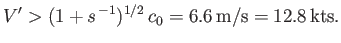(11.148)Next: Exercises Up: Waves in Incompressible Fluids Previous: Capillary Waves at an
Richard Fitzpatrick 2016-03-31